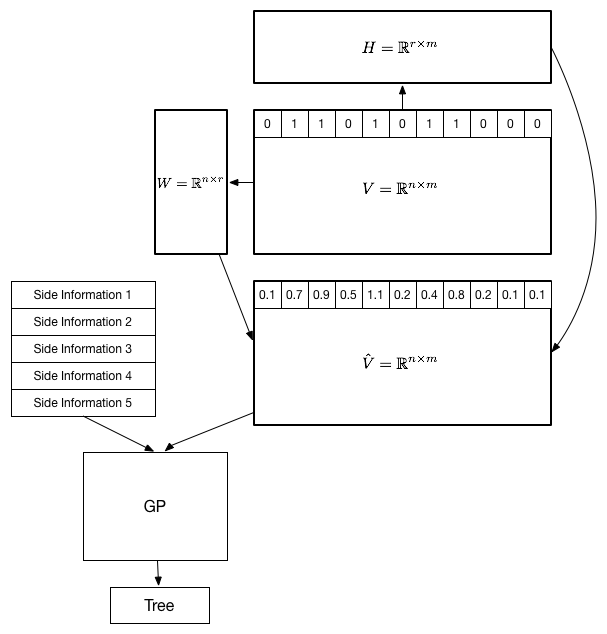# Sungjoo Ha

## Inspecting Latent Space with Genetic Programming

• Sungjoo Ha, Sangyeop Lee, and Byung-Ro Moon
• Genetic and Evolutionary Computation Conference, pp. 63-64, 2016
• [Paper]

We suggest a method of inspecting the latent space of stock market data using genetic programming. Given black box patterns and $\langle$stock, day$\rangle$ tuples a relation matrix is constructed. Applying a low-rank matrix factorization technique to the relation matrix induces a latent vector space. By manipulating the latent vector representations of black box patterns, the geometry of the latent space can be examined. Genetic programming constructs a tree representation corresponding to an arbitrary latent vector representation, allowing us to interpret the result of inspection.

Different model representations have different pros and cons. Therefore, it is desirable to devise a method of transforming a model representation into another that allows us to look at the model from a different angle.

In this paper, we explored a method of transforming a group of classifiers into vector representations using matrix factorization techniques. It is possible to transform this latent vector representation into a tree representation using genetic programming. This whole process can be understood as a transformation of a model representation into a tree representation.